# 2021年成人高考高起点《理数》模拟试题二

2021-03-03 15:38 江西成考网

1.A.5π/4
B.7π/4
C.π/4
D.-π/4

2.A.1/2 ,3
B.1/2 ,1
C.2,3
D.2,1

3.A.A
B.B
C.C.
D.D

4.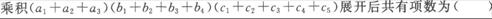A.12项
B.15项
C.20项
D.60项

5.函数y=2cos4x-2sin4x的最小正周期是

A.π
B.2π
C.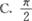D.4π

6.A.B.C.D.7.任选一个不大于20的正整数，它恰好是3的整数倍的概率是

A.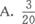B.C.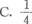D.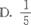8.f[f(x)]=x4-6x2+6，则f(x)为（　　）

A.f(x)=x2-3
B.f(x)=x2-12
C.f(x)=x2+6
D.f(x)=x2-6

9.A.B.C.D.10.A.1/9
B.2/9
C.8/45
D.16/45

11.A.B.C.D.12.A.3
B.4
C.5
D.6

13.A.x2+y2-1Ox+9=0
B.x2+y2-10x-9=0
C.x2+y2+10x-9=0
D.x2+y2+10x+9=O

14.下列命题中，正确的是

A.空间中，垂直于同一条直线的两条直线平行
B.空间中，垂直于同一平面的两条直线平行
C.空间中，垂直于同一平面的两平面平行
D.空间中，与同一平面所成角相等的两条直线平行

15.设定义域在R上的函数，f(x)=x|x|，则f(x)是

A.奇函数，增函数
B.偶函数，增函数
C.奇函数，减函数
D.偶函数，减函数

16.A.0＜a＜2/3
B.a＞2/3
C.2/3＜a＜1
D.0＜a＜2/3 或a＞1

17.A.a和b不可能垂直，也不可能平行
B.a和b不可能垂直，但可能平行
C.a和b可能垂直，但不可能平行
D.a和b可能垂直，也可能平行

18.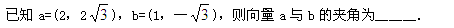19.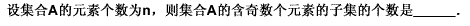20.21.22.已知在直三棱柱ABC-AlBlCl中，∠BAC=30°,∠ACB=90°,BC=1，AAl=(1)求证：平面AB1C1平面AA1C1C；
(2)求AB1与面AA1C1C所成角的正弦值；
(3)已知点M为CC1的中点，求A1M与AB1所成的角．

23.24.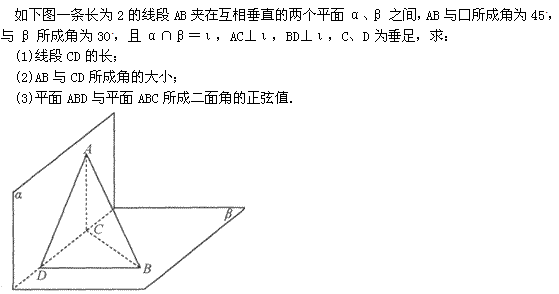710
2022年成人高考考试时间
10月15,16日+